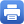Columbia Home

## Fall 2023

### MATH GR6263 – Topics in Algebraic Geometry

Instructor: John Morgan
Title: Differential Forms, Rational Homotopy Theory, and Smooth Complex Algebraic Varieties
Day/Time: TR 2:40PM – 3:55PM
Room: 507 Math
Abstract: The course will begin with a brief overview of homotopy theory including Postnikov Towers and localization in homotopy theory.  We will then introduce differential graded algebras (DGA’s) over the rationals and prove that these form a model for rational homotopy theory.  Lastly, the lectures will  develop and use the Kahler identities for differential forms on compact Kahler manifolds to study the rational homotopy theory of smooth Complex Algebraic Varieties, both projective and quasi-projective.

Course Prerequisites: The level of the course will be a second-year graduate course. We shall assume a basic understanding of (i) homotopy theory and  algebraic topology, including  homology and cohomology as well as the fundamental group, and (ii) differential topology including the basics of  differential forms on smooth manifolds. No knowledge of more advanced topics in these domains will  be assumed.

### MATH GR8507 – Topics in Topology

Instructor: Soren Galatius
Title: Topics in high-dimensional topology
Day/Time: MW 11:40AM – 12:55PM
Room: 507 Math
Abstract: This course will cover classical topics in high dimensional manifold theory, starting with the h- and s-cobordism theorems.  We will then discuss L-theory and surgery theory, roughly following parts of Wall’s book.  These classical results attempt to describe “structure sets” — sets of diffeomorphism classes of manifolds of a given homotopy type.  The rest of the course will discuss efforts to upgrade from structure sets to structure spaces.

The course should be accessible to PhD students familiar with the basics of differential topology and homotopy theory, for instance including the following concepts.
Differential Topology: smooth manifolds, embeddings, regular values and transversality, vector bundles.  Prior encounters with Morse functions helpful, but will not be assumed.
Homotopy Theory: homotopy groups, weak equivalence, homotopy fiber.  Prior encounters with simplicial sets helpful, but will not be assumed.Print this page